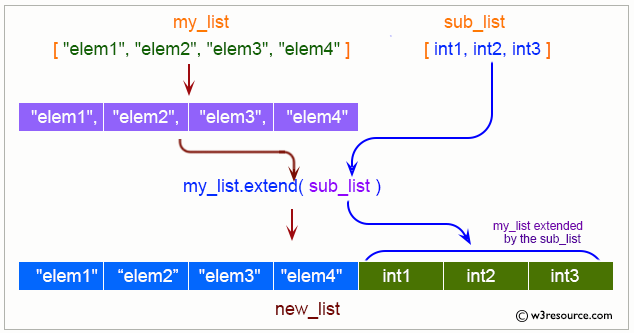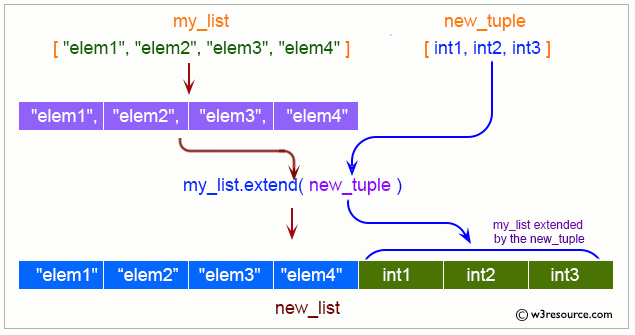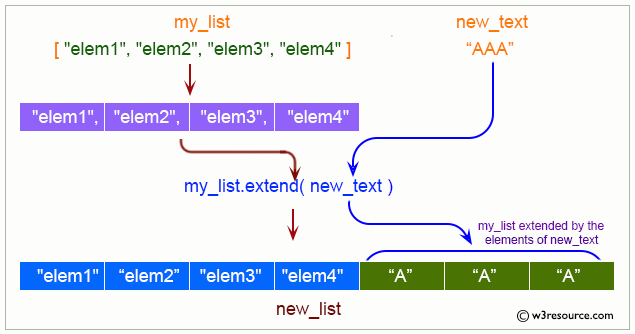# Python List: extend() Method

## extend() Method

The extend() method is used to extend a list by appending all the items from the iterable.

Visual Explanation:Syntax:

`list1.extend(iterable)`

Parameter:

• Required. Type -> any iterable (list, set, tuple, etc.)

Equivalent Command of extend() method:

`a[len(a):] = iterable`

Example 1: List extend() Method

``````
# colors list
color1 = ['Red', 'Green', 'Black']
print("First list:")
print(color1)
color2 = ['White', 'Orange']
print("Second list:")
print(color2)
print("Add the second list to the first list:")
color1.extend(color2)
print(color1)
``````

Output:

```First list:
['Red', 'Green', 'Black']
Second list:
['White', 'Orange']
Add the second list to the first list:
['Red', 'Green', 'Black', 'White', 'Orange']
```

Example 2: Add each element of a tuple to a list

``````
# colors list
colors = ['Red', 'Green', 'Black']
print("Original list:")
print(colors)
nums = (1, 2, 3, 4, 5)
print(nums)
print("Add each element of the said tuple to the colors list:")
colors.extend(nums)
print(colors)
``````

Output:

```Original list:
['Red', 'Green', 'Black']
(1, 2, 3, 4, 5)
Add each element of the said tuple to the colors list:
['Red', 'Green', 'Black', 1, 2, 3, 4, 5]
```

Example 3: Add each element of a string to a list

``````
# colors list
colors = ['Red', 'Green', 'Black']
print("Original list:")
print(colors)
text = "Pink"
print(text)
print("Add each element of the said string to the colors list:")
colors.extend(text)
print(colors)
``````

Output:

```Original list:
['Red', 'Green', 'Black']
Pink
Add each element of the said string to the colors list:
['Red', 'Green', 'Black', 'P', 'i', 'n', 'k']
```

Python Code Editor:

Previous: Python List count() Method.
Next: Python List index() Method.

Test your Python skills with w3resource's quiz

﻿